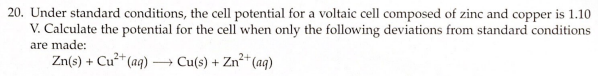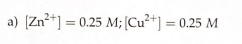# 20. Under standard conditions, the cell potential for a voltaic cell composed of zinc and copper is 1.10 V. Calculate the potential for the cell when only the following deviations from standard conditions are made: Zn(s) + Cu^2+(aq) → Cu(s) + Zn^2+(aq) a) [Zn^2+] = 0.25 M; [Cu^2+] = 0.25 M